PHYSICS

УДК 539

Corresponding Member of NAS RA A. G. Bagdoev, A. V. Shekoyan

Generalized Non-linear Equations of Magnetohydrodynamical Media

(Submitted 17/III 2006)

Keywords: Maxwell equations, magnetohydrodynamics, Galilean transformations

From elder classes of school we were fascinating by the theory of relativity, which we studied by popular literature.
At the ripe age the sceptical attitude to the theory of relativity arised in ourselves. In the middle of 60 th of XX th century A.G. Bagdoev informed the president of AS of Armenia V. A. Ambartsumyan, and some years ago also the leading Armenian physicists about his judgments on this question.
It is difficult to say that they completely agreed with him, but there weren't also any remarks very much to the point. Recently we acquainted with the works of Academician of RAS S.S Grigoryan , where he showed insolvency of the general theory of relativity in explanation of various Cosmogonic problems, in particular, of generation of the black holes, and on the other hand he showed the applicability of the Newton's theory, developed by Mitchell and Laplace to this problems. This encouraged us to write this article, concerning to the critical analysis of the principal sources of the special theory of relativity. There are experimental data of American physicists from Los-Alamos about variability of the speed of light and facts of constancy in big volumes of temperature in cosmic objects, which leads to the necessity to take into account the fact of the presence of the super light speed. These facts, which are in contradiction with special theory of relativity, are brought in the article of Pyotr Obraztov, published "In science world" N2, 2005.
The basic argument for creation the special theory of relativity was the thesis due to which the linear Maxwell equations are invariant with respect to more general Lorents transformations, in contrast to non-linear equations of mechanics, which are invariant with respect to Galilean transformations and therefore one must fit the equations of mechanics to these more general transformations.
Following to this logics one must prefer to general non-linear equations of hydromechanics, which are invariant with respect to Galilean transformation, the obtained from them after linearisation for small disturbances, wave equation, which is invariant with respect to more general Lorents transformation. Of course nobody will insist on it. Then the question arises whether it is not better to fit Maxwell equations, derived in earth conditions for relatively small electromagnetic fields with respect to the enorme light speed, and write down non-linear Maxwell equations, which by analogy with mentioned example, must satisfy to more particular but more natural Galilean transformations.
By the way the equations of this type are equations of magnetohydrodynamics, obtained from matching solution of Maxwell equations for moving media [2, 3]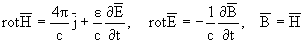(1)
where current density is connected with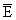by Ohm law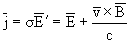(2)
with equations of motion of media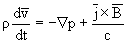(3)
For not too small variated in time processes or (which is the same) for not too large frequencies, the item with displacement current in (1) can be neglected, and as a result the induction equation can be obtained from (1), (2)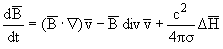(4)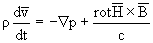(5)

and equations (4), (5) are invariant with respect to Galilean transformation, where to the same accurancy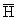can be taken as the invariant.
These equations were successfully applied to study non-linear waves in pulsars [4, 5], where the great frequencies and large magnetic fields take place. In these conditions the Alfen velocity has the same order as light velocity. In such problems we must take into account non-linear effects and solve non-linear Maxwell equations coupled with hydrodynamical equations Usually in magnetohydrodynamics frequencies of process w are much smaller than electroconductivity s, which allows to neglect the displacement current.
In the case of w ~ s for example for magnetic stars [4, 5] w ~ 1014[1/sec], we have also to take into account the displacement currents in magnetohydrodynamic equations and, as it was mentioned, to take into account non-linearity for considered great magnetic fields, i.e. to write down non-linear Maxwell equations.
As an example of taking into account the displacement current in non-linear equations (1)-(3) which satisfy the condition of invariancy with respect to the Galilean transformations, as well as in (4), (5) we can replace the displacement current in (1) on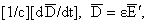where derivative in time is taken for moving particle. Then system (1)-(3) again will be invariant respectively to Galilean transformations, where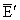is a simply parameter, which can be excluded as in derivation of (4).
Taking into consideration the improved value of displacement current we have equations of moving electroconducting media in the form (1)-(3), where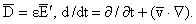Note that taking into account the displacement current demands to revise orders of parameters. To do it let us simplify the system of equations, making the iteration in the first equation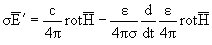(6)
Placing (6) in (2) we obtain the induction equation with the accounting of displacement current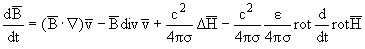(7)
Here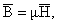m = 1. Taking into consideration that in propagating waves [d /dt] ~ w, С ~ [(w)/(cn)], where cn is wave speed, from (7) we obtain orders w ~ s,
 v ~ cn,   cn ~ c,    E ~ H
(8)
where [3, 4] cn ~ a1, a1 =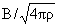is Alfen speed. From the equation of motion (3) we obtain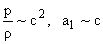(9)
It is evident that orders (8), (9) take place for general nonsimplified system of equations as well.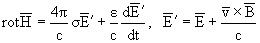(10)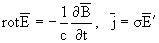(11)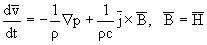(12)

System (10)-(12) due to (8), (9) describes the motion of electroconducting fluids in great magnetic fields and with great velocities and pressures, and is invariant with respect to Galilean transformation.
Of course it must be considered only as a possible example of modification of Maxwell equations for moving media, and accounting of non-linearity, the mentioned equations are invariant with respect to Galilean transformations.
Note that system (10)-(12) is closed together with the equation of continuity of mass and the state equation of fluid. At the same time it takes place the  equation of continuity of charge density re in form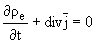(13)

If we place a usual Maxwell equation (1) due to which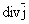= -[1/(4p)][()/(t)]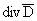in (13), we obtain from (13)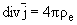(14)
which is the known Culon law, derived initially for static problem, and postulated by Maxwell for dynamic problems, by the way, for inmoved media. In considered statement of problem for moving media equations (10)-(12) yield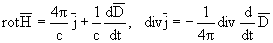(15)
From (13) and (15) we obtain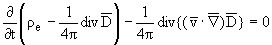(16)

After resolving the equations (10)-(12), from (16) we obtain re.
For inmoving media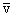= 0 and (16) coincides with (14), and in general case of moving media the correctness of (16) is not less based than of equation (14). Also note that as it is mentioned in  it is not necessary to derive (2) from Lorents transformations, in which the velocity of inertial system is supposed to be constant, but in (2)is a variable velocity of particle, and as it is done in  to assume (2) as an experimental Ohm law for moving media. Thus, on our opinion, the special theory of relativity can be considered as an asymptotic theory taking place for large velocities and bounded fields, which allows it to describe the motion of elementary particles with corresponding shortening of length and time, but does not make a fetish of its results in application to non-linear equations of mechanics, as well as to electrodynamic equations, particularly for large electromagnetic fields.
In applications to these non-linear equations Galilean transformations are more natural than more general by form, but more particular by applications, Lorents transformations.
Also note that equations of motion of two-component plasma and equations of motion of mixtures of gas and fluid in clouds are not invariant with respect to Lorents transformation, and for the former ones the dispersion law for waves is obtained, which gives electromagnetic waves for large frequencies and magnetohydrodynamic waves for small ones .
And it is not posed the condition of invariancy of equations with respect to any transformations in inertial systems, which is the basic argument of the special theory of relativity.

Institute of Mechanics NAS RA

Литература

1. Григорян С.С. В сб.: Проблемы динамики взаимодействия деформируемых сред. V Междунар. конф. Горис. 2005. С. 164-169.
2. Кугушев А.М., Голубева Н.С. Основы радиоэлектр. М. Энергия. 1969. 879 с.
3. Багдоев А.Г., Седракян Д.М. - Астрофизика. 2002. Т.45. Вып. 1. С. 63-68.
4. Багдоев А.Г., Седракян Д.М. - Астрофизика. 2003. Т.46. Вып. 1. С. 87-94.
5. Тамм И.Е. - Основы теории электричества. М. Наука. 1966. 624 с.
6. Франк-Каменецкий Д.А. Лекции по физике плазмы. М. Атомиздат. 1964. 283с.